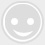# 单片机音乐中音调和节拍的确定方法,可以试着去玩玩!

-回复 -浏览A的频率f = 440 Hz,其对应的周期为：

T = 1/ f = 1/440 =2272μs

t = T/2 = 2272/2 = 1136μs

t = 12 *(TALL – THL)/ f0

TH = THL / 256 = ( TALL – t* f0/12) / 256

TL = THL % 256 = ( TALL – t* f0/12) %256

TH440Hz = (65536 – 1136 * 12/12) /256 = FBH

TL440Hz = (65536 – 1136 * 12/12)%256 = 90H

xueshiran@buaacm.com.cn

3.如何让嵌入式软件的复杂电子设备更便宜、更可靠？

4.张忠谋：芯片产业已成熟，年轻工程师可向软件方面谋发展

6.做技术的都要想想这个问题：商业模式创新比技术创新更重要吗？